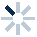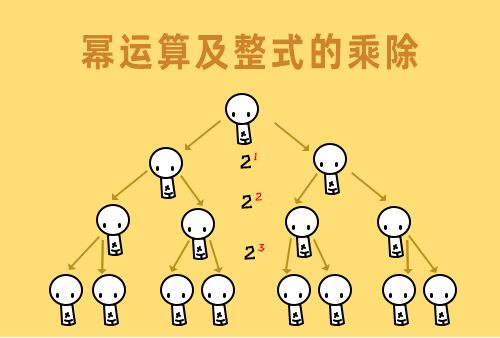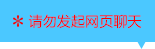| | |

||
APP端下载AndroidiPhone
|
￥85

|
2455人点赞
213746人已学习
|

3天无理由退款

3天无理由退款：退款将以超级币形式退至您的超级课堂学习账户，便于您重新选购其他课程。恶意退款将被冻结账号。

• 1、正弦就是对边比斜边，余弦就是邻边比斜边，正切就是对边比邻边。
2、 三角函数就是一种以锐角为自变量，以比值为函数值的函数。三角函数值就是一个比例。
• 1、我们介绍了简单的三角函数值的求法。
2、 知道直角三角形中的任意两边，或者任意两边的比例，或者任意一个三角函数值，都能利用勾股定理，把每种三角函数值都求出来。
• 1、我们介绍了如何在给定图形中求某个角的三角函数值。
2、 必须在直角三角形里求，如果所求的角并不在直角三角形里，可以采取构造法或角度替换法来解决。
• 1、记住三种特殊角的三角函数值：$sin30^{\circ} 2、 =\dfrac{1}{2}$，$cos30^{\circ} =\dfrac{\sqrt{3}}{2}$，$tan30^{\circ}=\dfrac{\sqrt{3}}{3}$。$sin45^{\circ} 3、 4、 =\dfrac{\sqrt{2}}{2}$，$cos45^{\circ} =\dfrac{\sqrt{2}}{2}$，$tan45^{\circ}=1$。$sin60^{\circ} 5、 6、 =\dfrac{\sqrt{3}}{2}$，$cos60^{\circ} =\dfrac{1}{2}$，$tan60^{\circ}=\sqrt{3}$。
7、 这节课最重要的还是在于记忆，对三大奇葩特殊角，和它们对应的三组函数值，有充分的熟悉，这点对于你以后快速解题，找到边长角度关系，有着决定性意义，后面的内容可是分分钟都要用到这些知识点哦。
• 1、我们介绍了角度和三角函数值的“一一对应”关系。
2、 在锐角范围内，一个角对一个值，一个值也对一个角。所以可以通过特殊角得到特殊值，也可以通过特殊值得到特殊角
• 1、我们介绍了如何利用构造法，求15度和75度的三角函数值。
2、 15度与75度角并不常用，它们的三角函数值只需了解就好，不需要记住，这种构造的思想其实比函数值更加重要，需要你好好体会。
• 1、​同角三角函数的平方关系：$sin^{2}\alpha +cos^{2}\alpha =1$。
2、 利用平方关系，可以实现正弦与余弦的互化。
• 1、​利用平方关系解题，蕴藏着一大技巧，那就是正弦、余弦的和、差、积，知一求二。
• 1、​我们介绍了一些利用平方关系解题的实用技巧，比如对已知式子进行变形，采用整体思想代入求解。
• 1、同角三角函数的商数关系：$tan\alpha =\dfrac{sin\alpha }{cos\alpha }$。
2、 利用商数关系，可以实现“弦化切”。
3、 含有三角函数的式子，对$1$要灵活处理，经常利用平方关系，化身为$sin^{2}\alpha +cos^{2}\alpha$。
• 1、关于互余两角的三角函数关系，要记住三个基本公式$sin(90^{\circ}-\alpha )=cos\alpha ^{2}$，$cos(90^{\circ}-\alpha )=sin\alpha ^{3}$，$tan(90^{\circ}-\alpha )=\frac{1}{tan\alpha }$。
• 1、​互余两角的三角函数关系和平方关系联系在一起又推出了两个新的公式。$sin^{2}\alpha +sin^{2}（90^{\circ}-\alpha ）=1$和$cos^{2}\alpha +cos^{2}（90^{\circ}-\alpha ）=1$，记为互余两角正弦或余弦的平方和都是$1$。
2、 还通过例题向你展示了分组思想的巧妙，你要对和为$90^{\circ}$的一对角度特别敏感，顺利的完成配对。
3、 到这里，初中三角函数的基本关系网就全部介绍完毕了，我们可以把这几节课的关系图拼在一起，所有这些公式都是需要你非常熟悉，能够记住的，三角函数圈的那些事儿其实就这么清晰！
• 1、三角函数的有界性确定了三角函数值整体的范围：$0＜ sin\alpha ＜ 1,0＜ cos\alpha ＜ 1,tan\alpha ＞0$。我们通过它来验证所求值是否可能成为三角函数值。
2、 增减性确定了在锐角范围内，$sin\alpha$和$tan\alpha$会随着$\alpha$的增大而增大，而$cos\alpha$会随着$\alpha$的增大而减小。
3、 各种不同的题型，最终都只利用了三角函数的两大性质，有界性和增减性，你可要彻底记住哦！
• 1、主要内容是三角函数增减性的应用一和应用二。
2、 应用一：同一种三角函数，不同角之间的大小比较。对于$sin\alpha$和$tan\alpha$，角越大则值越大，值越大则角越大；对于$cos\alpha$，角越大则值越小，值越大则角越小。
3、 应用二：同一个角度，不同三角函数之间的大小比较。（1）比较$sin\alpha$和$cos\alpha$：当$\alpha ＜45^{\circ}$时，$sin\alpha ＜cos\alpha$；当$\alpha ＞45^{\circ}$时$sin\alpha ＞cos\alpha$；当$\alpha =45^{\circ}$时，$sin\alpha =cos\alpha$。（2）比较$sin\alpha$和$tan\alpha$：在锐角范围内$sin\alpha$始终小于$tan\alpha$。
• 1、主要内容是三角函数增减性的应用三。
2、 应用三：确定范围，根据角的范围确定三角函数值的范围，或根据三角函数值的范围确定角的范围。需要你牢记三种特殊角的三角函数值，由角到值就要添函数名，由值到角就要去函数名。要注意对于$sin\alpha$和$cos\alpha$的变化不用变号，$cos\alpha$的变化需要变号。
• 1、解直角三角形的概念是，在直角三角形中，由已知元素求未知元素的过程。它遵循知二求三的原则，包含两种情况：已知两边或已知一边一角。由边求边用勾股，由角求角用互余，边角互求用三角函数。这就是解直角三角形的依据。
2、 要记住三组关系，一组是$a=csinA$和$b=csinB$，简记为“直角边等于斜边乘以对角的正弦”。第二组是$a=ccosB$和$b=ccosA$，简记为“直角边等于斜边乘以邻角的余弦”。第三组是$a=btanA$和$b=atanB$，简记为“直角边等于另一直角边乘以对角的正切”。
• 1、​我们介绍了边角关系知二求三在题目中的具体应用。
2、 这节课还是在帮你熟悉各个三角函数的定义，通过角度对应的三角函数值，确定边长的比例关系，解出直角三角形的每一个元素。
• 1、我们认识了三角函数的一种题型——三角形嵌套模型。在利用三角函数求复杂图形的边长时，要注意将条件凑到一个直角三角形中。
• 1、我们认识了三角函数的第二种题型——圆上解直角三角形，要学会利用直径构造直角三角形，利用圆周角定理进行角的转化。
• 1、要确定一个一般三角形的形状，至少要知道三个元素，而且至少要知道一条边的长度。
2、 作高分割三角形的第一类常见题型是已知两角一边，可分为两角+对边和两角+夹边。
3、 已知两角一边的情况时的解题步骤。首先过未知角的顶点作高，将图形分成两个直角三角形。然后利用作为公共边的高，去解第二个直角三角形，或者建立方程。
• 1、我们介绍了作高解一般三角形的第二类常见题型——已知两边一角，可分为两边+夹角和两边+对角。
2、 当已知两边+夹角时，过未知角的顶点作高，分成两个直角三角形；当已知两边+对角时，将已知的两边分别作为两个直角三角形的斜边，但要注意分类讨论。
• 1、作高的技巧是，过未知角的顶角作高，在作高时要保留已知角。而且除了已知两边+夹角的情况，尽量保留已知边，充分利用所给的边、角条件。唯一需要注意的是两边+对角的题型，有两种情况，要分类讨论。
2、 讲解一道较难的题目，要注意画图，当无法直接求出边长时，可以选择尝试用勾股定理列方程。
• 1、三角形的面积公式$S=\frac{1}{2absin\alpha }$，即任意两边的乘积乘以它们夹角的正弦除以$2$。
2、 当$\angle C$为直角时，$sinC$取$1$。
3、 当$\angle C$为钝角时，$C$取$\angle C$的补角。
• 1、三角形的面积公式$S=\dfrac{1}{2}absinC$ ，除了计算面积，在证明题当中，也有妙用。
• 1、​平行四边形的面积公式$S=absin\alpha$积乘以它们夹角的正弦。若$\alpha$是直角，则$sin\alpha$取$1$；若$\alpha$是钝角，则$sin\alpha$取$sin(180^{\circ}-\alpha )$。
2、 四边形的面积公式为$S=\dfrac{1}{2}absin\theta$ 。即四边形的面积等于对角线乘积乘以它们夹角的正弦除以$2$。当对角线垂直时，$sin\theta$取$1$，$S=\dfrac{1}{2}ab$。
• 1、在测量时，视线与水平线所成的角中，视线在水平线上方的叫仰角，视线在水平线下方的叫俯角。
• 1、关于解决实际测量应用题，总结了两种基本图形，与各自旋转$90$度后的变形。
2、 基本图形1推导出的公式为$b=a\cdot \dfrac{tan\alpha \cdot tan\beta }{tan\beta -tan\alpha}$
3、 基本图形2推导的出的公式为$b=a\cdot \dfrac{tan\alpha \cdot tan\beta }{tan\beta + tan\alpha }$。
• 1、当测量点不在地面时，不要忘记把人或仪器的高度加上去。
2、 最后是一种基本图形的变相应用，通过辅助线来构造基本图形。
3、 利用这节课的技巧，再配上一台能测量仰俯角的测绘仪器，你就完成了测绘学的入门课程。不管是摩天大楼，还是高山之巅，通过基本图形和公式，就没有你求不出的高度。
• 1、坡面的铅直高度$h$和水平宽度l的比叫做坡面的坡度，也叫坡比，一般用$i$来表示，坡面与水平面的夹角$\alpha$叫做坡角。坡度是坡角的正切，即$i=tan\alpha$。
2、 坡度也可以用任意两点垂直距离与水平距离的比来表示。坡面距离，垂直距离和水平距离三者会构成此类应用题中的直角三角形，直接运用三角函数解决。
• 1、我们学习了坡面上的影长问题。光线、物体和影子构成三角形，如果物体在平坡上则构成四边形，就要作辅助线将四边形拆分为两个特殊三角形，再来求相关边长。
• 1、方向角的定义：以观测者的位置为中心，将正北或正南方向线旋转到目标的方向线所成的角。
2、 要熟悉正北、正南、正东、正西，以及东北、东南、西北和西南所代表的方向角。
• 1、方向角问题，实质依然是解直角三角形，“作高”是最常用的手段，测得的方向角都可以直接或间接地转化到所解的三角形内。
• 1、对于有些高难度的方向角题目，没有直接给出边长，这时可以选择设元法列方程，利用勾股定理解出需要的长度。
2、 出海归来，我们来概括一下解直应用系列课程的内容，仰角俯角问题、坡度坡角问题和方向角问题，都是把实际问题转化成数学问题，这种转化包括两个方面：一是将实际问题的图形转化为几何图形，画出正确的平面或截面示意图，二是将已知条件转化为示意图中的边、角或它们之间的关系。在解三角形时，如果示意图不是直角三角形，要添加适当的辅助线，构造出直角三角形。
• 解直角三角形综合练习

• 1Enthu
• 2蒙阴第一深
• 3超级学员3900715
• 4超级学员4148773
• 5hissy
• 6超级学员4174990
• 7超级学员4201246
• 8超级学员4222001
• 9超级学员4228561215809人在学
￥ 88 ￥ 88265705人在学
￥ 12 ￥ 12214460人在学
￥ 88 ￥ 88214760人在学
￥ 63 ￥ 63

• 0
• 点击分享有好礼
••app端下载关注微信号